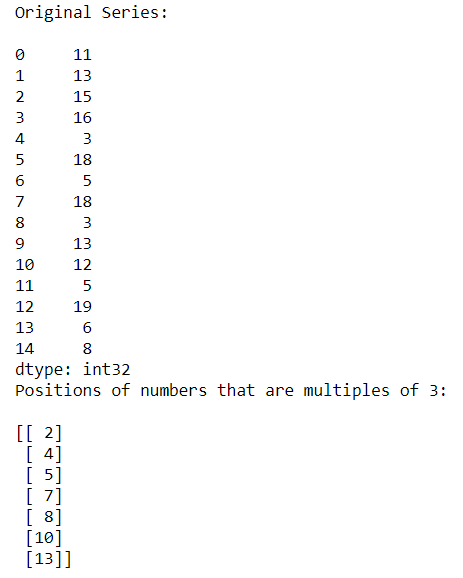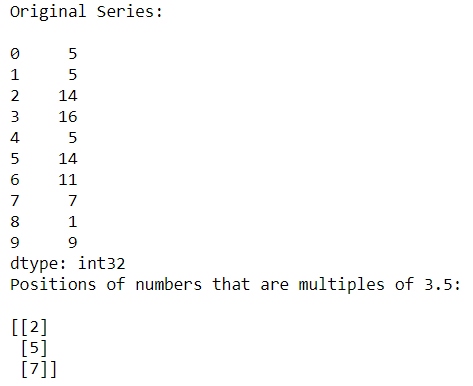# Find the position of number that is multiple of certain number

• Last Updated : 11 Oct, 2020

In this type of question, a number is given and we have to find the position or index of all multiples of that number. For doing this question we use a function named numpy.argwhere().

Syntax:

```numpy.argwhere(array)
```

Example 1:

Sometimes we need to find the indexes of the element that are divisible by int or float number.

## Python3

 `# Importing Pandas and Numpy libraries ` `import` `pandas as pd ` `import` `numpy as np ` ` `  `# Creating a Series of random numbers ` `n_series ``=` `pd.Series(np.random.randint(``1``, ``25``, ``15``)) ` `print``(``"Original Series:\n"``) ` `print``(n_series) ` ` `  `# Finding the indexes of numbers divisible by 3 ` `res_index ``=` `np.argwhere(n_series ``%` `3``=``=``0``) ` `print``(``"Positions of numbers that are multiples of 3:\n"``) ` `print``(res_index)`

Output:In the above example, we find the indexes of all numbers divisible by 3.

Example 2:

## Python3

 `# Importing Pandas and Numpy libraries ` `import` `pandas as pd ` `import` `numpy as np ` ` `  `# Creating a Series of random numbers ` `n_series ``=` `pd.Series(np.random.randint(``1``, ``20``, ``10``)) ` `print``(``"Original Series:\n"``) ` `print``(n_series) ` ` `  `# Finding the indexes of numbers divisible by 3.5 ` `res_index ``=` `np.argwhere(n_series ``%` `3.5``=``=``0``) ` `print``(``"Positions of numbers that are multiples of 3.5:\n"``) ` `print``(res_index)`

Output:In the above example, we find the indexes of all numbers divisible by floating-point number 3.5

My Personal Notes arrow_drop_up
Recommended Articles
Page :